APP Information

 Download Version 1.2 (403) Apk Size 8.66 MB App Developer Apptic Lab Malware Check UNKNOWN Install on Android 4.0.x and up App Package com.nkus.zolo_plus.apk MD5 9eff777a65e12079cfacac7c3b6344eb Rate 5### App Description

```The Calculator provides the following features:
ALGEBRA
1. Computer science
2. Solving the equation:
3. Solve system equations
4. Graphing
5. Cartesian geometry sand(Viet Nam Education)
6. Unit Conversions
8. Simplify expressions
9. Polynomial factorization.
10. Binomial expansion Newton
11. Matrix: evalute matrix step by step
ANALYTICS
1. Derivative
2. Antiderivative
3. Definite integrals
4. Find the limit of the sequence, function
TRIGONOMETRIC
1, Trigonometric expand: sin(2x) -> 2sin(x)cos(x)
2. Trigonometric reduce: 2sin(x)cos(x) -> sin(2x)
3. Trigonometric to exponent: sinh(x) -> (e^x-e^(-x))/2
DETERMINATION OF STATISTICS
1. Combination
2. Permutation
SOME OTHER FEATURES
1. Prime factors
2. Modulo
3. Catalan number
4. Fibonacci number
The Calculator does not show the steps to solve the equations, system of equations, derivative... but only for the final result.
Enter sin(30°) to calculate sine Degree mode
The computer can work in two modes:
- Decimal mode: 0.12312312323
- Mode fractions: for results with arbitrary precision, for example 9^99999
Any suggestions on the application please send mail: [email protected] ```

### App ChangeLog

• Unit Converter⭐️
• Calculator⚡️
• Graph📉
• Bugs Fixed✨

### More Android Apps to Consider

1.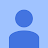Great app ever Used! Best Math Solver App!

2.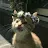NO ONE

This app made my life easier, Thanks for creating this app 🥺😍

3.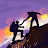Ottoman Alee

Best App Ever Used! Great

4.Komal Tariq

Loved this app

5.Mishaal Riaz

Good app

6.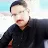Cool app

7.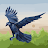Ali asghar

Nice app

8.9.10.